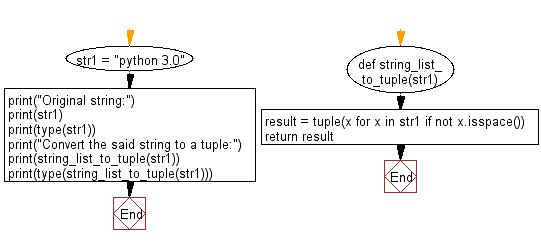﻿ Python: Convert a given string list to a tuple - w3resource# Python Exercise: Convert a given string list to a tuple

## Python tuple: Exercise-25 with Solution

Write a Python program convert a given string list to a tuple.

Sample Solution:-

Python Code:

``````def string_list_to_tuple(str1):
result = tuple(x for x in str1 if not x.isspace())
return result
str1 = "python 3.0"
print("Original string:")
print(str1)
print(type(str1))
print("Convert the said string to a tuple:")
print(string_list_to_tuple(str1))
print(type(string_list_to_tuple(str1)))
```
```

Sample Output:

```Original string:
python 3.0
<class 'str'>
Convert the said string to a tuple:
('p', 'y', 't', 'h', 'o', 'n', '3', '.', '0')
<class 'tuple'>
```

Flowchart:## Visualize Python code execution:

The following tool visualize what the computer is doing step-by-step as it executes the said program:

Python Code Editor:

Have another way to solve this solution? Contribute your code (and comments) through Disqus.

What is the difficulty level of this exercise?

Test your Programming skills with w3resource's quiz.

﻿

## Python: Tips of the Day

Decapitalizes the first letter of a string:

Example:

```def tips_decapitalize(s, upper_rest=False):
return s[:1].lower() + (s[1:].upper() if upper_rest else s[1:])
print(tips_decapitalize('PythonTips'))
print(tips_decapitalize('PythonTips', True))
```

Output:

```pythonTips
pYTHONTIPS
```